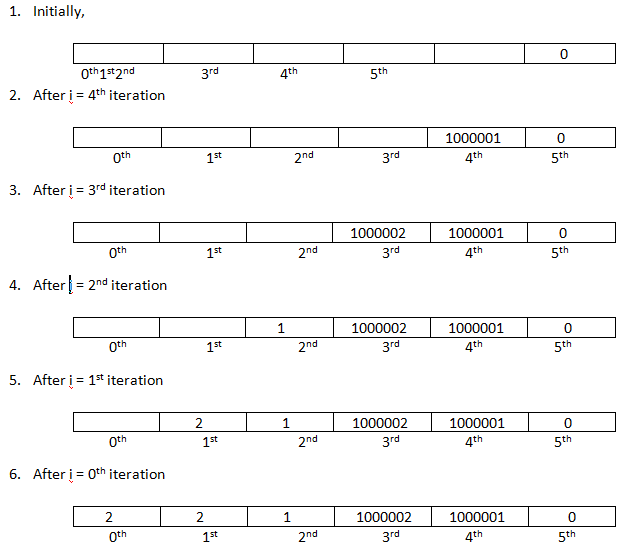# Minimum jumps required using Dynamic programming (DP)

Given an array of integers of length N in which value of each index represent maximum jump u can take from that index. You have to find minimum jumps required to reach from 0th index to the end of the array(last index).
Submitted by Ritik Aggarwal, on December 17, 2018

Problem: You are given an array of integers of length N in which value of each index represents maximum jump u can take from that index. You have to find minimum jumps required to reach from 0th index to the end of the array(last index).

Constraints:

```    1 <= N <=  30,000
0 <= A[i] <= 100
```
```    Sample Input 1:
6
2 2 3 1 0 3
Sample Output 1:
minimum jumps required is 2

Sample Input 2:
11
1 3 5 8 9 2 6 7 6 8 9
Sample Output 2:
minimum jumps required is 3
```

Explanation of the problem:

For the sample Input1,

We can jump from 0th index to 2nd index then to last index. So, 2 jumps required.

For the sample input2,

We can jump from 0th index to 1st index then 3rd index and then last index. So, 3 jumps required.

Solution: For sample input – 1

STATUS OF DP MATRIX AFTER ith ITERATIONNote: Since, we have to take minimum of all the possible jumps so if there are no jumps possible that is arr[i] = 0 then cell will get initialize to non- updated minimum value that is 1000000(denotes infinite steps). Due to presence of 1000001 at 4th index 3rd index also gets this value though arr is non-zero.

Algorithm:

• STEP-1: Create a 1D dp matrix in which ith cell represent minimum jumps required from ith index to the last.
• STEP-2: Initialize the last index of dp matrix to be zero as it is the end of the array.
• STEP-3: Start filling the dp matrix from second last index.
• STEP-4: From ith index we can jump maximum of arr[i] value so taking minimum of dp[i + j] where j is ranging from 1 to arr[i] and i + j must be less than length of dp matrix.
• STEP-5: Add 1 to the answer got from step 4(1 jump is required from ith to i+j index) and store the answer at ith index of dp matrix.
• STEP-6: return the answer of 0th index of dp matrix.

The time complexity of the above code is O(N^2).

C++ Implementation

```#include <iostream>
using namespace std;

int minJumps(int arr[], int n){
int dp[n] = {0};
// if already at last index the number of steps is 0
dp[n-1] = 0;
// filling from second last index
for(int i = n - 2;i>=0;i--){
int min = 1000000;
// value at an index of arr donates maximum_possible jump
int jumps_range = arr[i];
// i + j < n is used to avoid jumping out of array length
// && also jump can vary from 1 to value of arr at that index
for(int j = 1;j<=jumps_range && i + j < n;j++){
// taking minimum of all possible jumps as ith index of
// dp matrix represent minmum jumps required to reach from ith
// index to the end
if(min > dp[i+j]){
min = dp[i+j];
}
}
// also have to add 1 as 1 jump is required to jump on any
// index in jump_range and storing that value
dp[i] = 1 + min;
}
// return the minimum jumps from 0th index to the end
return dp;
}

// driver function to check the code
int main() {
int n;
cout<<"Enter size of the array: ";
cin >> n;
int arr[n] = {0};

cout<<"Enter array elements: ";
for(int j = 0;j<n;j++){
cin >> arr[j];
}
for(int val : arr){
cout << val << " ";
}
cout <<"\nminimum jumps required is "<< minJumps(arr, n) << endl;
return 0;
}
```

Output

```Enter size of the array: 6
Enter array elements: 2 2 3 1 0 3
2 2 3 1 0 3
minimum jumps required is 2
```

What's New

Top Interview Coding Problems/Challenges!

Languages: » C » C++ » C++ STL » Java » Data Structure » C#.Net » Android » Kotlin » SQL
Web Technologies: » PHP » Python » JavaScript » CSS » Ajax » Node.js » Web programming/HTML
Solved programs: » C » C++ » DS » Java » C#
Aptitude que. & ans.: » C » C++ » Java » DBMS
Interview que. & ans.: » C » Embedded C » Java » SEO » HR
CS Subjects: » CS Basics » O.S. » Networks » DBMS » Embedded Systems » Cloud Computing
» Machine learning » CS Organizations » Linux » DOS
More: » Articles » Puzzles » News/Updates